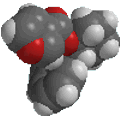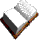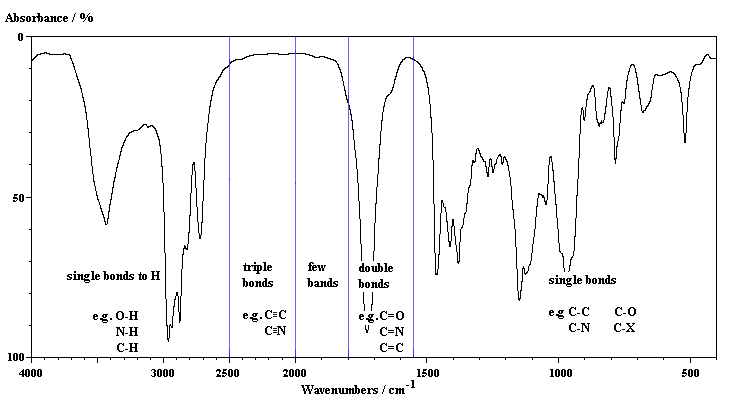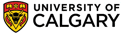Chapter 13: SpectroscopyInfra-Red (IR) Spectroscopy

 Hookes' LawTo help understand IR, it is useful to compare a vibrating bond to the physical model of a vibrating spring system.  The spring system can be described by Hooke's Law, as shown in the equation given on the left.  Consider a bond and the connected atoms to be a spring with two masses attached. Using the force constant k (which reflects the stiffness of the spring) and the two masses m1 and m2, then the equation indicates how the frequency, u, of the absorption should change as the properties of the system change.

Consider the following trends:

1. for a stronger bond (larger k value), u increases.
As examples of this, in order of increasing bond strength compare:
CC bonds: C-C (1000 cm-1), C=C (1600 cm-1) and C≡C (2200 cm-1),
CH bonds: C-C-H (2900 cm-1), C=C-H (3100 cm-1) and C≡C-H (3300 cm-1),
(n.b. make sure that you understand the bond strengths order)
2.  for heavier atoms attached (larger m value), u decreases.
As examples of this, in order of increasing reduced mass compare:
C-H  (3000 cm-1)
C-C  (1000 cm-1)
C-Cl (800 cm-1)
C-Br (550 cm-1)© Dr. Ian Hunt, Department of Chemistry